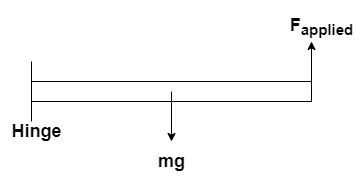# Problem: A uniform 300-N trapdoor in a ceiling is hinged at one side.a) Find the net upward force needed to begin to open it, if the upward force is applied at the center of the edge opposite the hinges.b) Find the total force exerted on the door by the hinges, if the upward force is applied at the center of the edge opposite the hinges.

###### FREE Expert Solution

Torque:

$\overline{){\mathbf{\tau }}{\mathbf{=}}{\mathbf{r}}{\mathbf{F}}{\mathbf{s}}{\mathbf{i}}{\mathbf{n}}{\mathbf{\theta }}}$

Newton's second law:

$\overline{){\mathbf{\Sigma F}}{\mathbf{=}}{\mathbf{ma}}}$

Let the length of the door be L.(a)

The force applied acts at a distance L from the rotational point while the weight acts at L/2 from the hinges.

The forces and radial distance are perpendicular — θ = 90°

ΣT = 0

(L/2)•mgsin 90 - (L)Fapplied sin 90 = 0

85% (322 ratings)###### Problem Details

A uniform 300-N trapdoor in a ceiling is hinged at one side.

a) Find the net upward force needed to begin to open it, if the upward force is applied at the center of the edge opposite the hinges.

b) Find the total force exerted on the door by the hinges, if the upward force is applied at the center of the edge opposite the hinges.